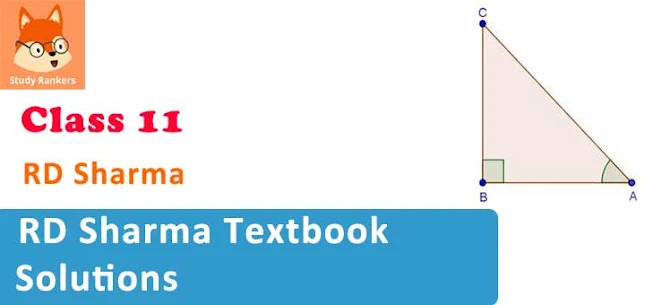# RD Sharma Textbook Solutions for Class 11 Maths

RD Sharma Solutions for Class 11 Maths is very helpful in building key concepts in the students. These RD Sharma Textbook solutions are prepared by our experts. Solutions provided on this website is solved stepwise so that it became easy for students to understand. We have also solved illustrative examples and MCQ provided in the textbook. A complete solutions to RD Sharma class 11 Maths is given on this website.Features of RD Sharma Solutions for Class 11 Maths:
• Solutions are provided stepwise.
• Complete solutions are given with indicative formulas.
• Easy way to solve problems along with concepts.
• Detailed explanations to each and every questions.
Class 11 RD Sharma Solutions is free resource which can be studied by students to achieve growth in their life. The lessons in RD Sharma is designed in such a way that it boost the knowledge and confidence.

## Chapterwise RD Solutions for Class 11 Maths

• Chapter 1 Sets
• Chapter 2 Relations
• Chapter 3 Functions
• Chapter 4 Measurement of Angles
• Chapter 5 Trigonometric Functions
• Chapter 6 Graphs of Trigonometric Functions
• Chapter 7 Trigonometric Ratios of Compound Angles
• Chapter 8 Transformation Formulae
• Chapter 9 Trigonometric Ratios of Multiple and Submultiple Angles
• Chapter 10 Sine and Cosine Formulae and Their Applications
• Chapter 11 Trigonometric Equations
• Chapter 12 Mathematical Induction
• Chapter 13 Complex Numbers
• Chapter 15 Linear Inequations
• Chapter 16 Permutations
• Chapter 17 Combinations
• Chapter 18 Binomial Theorem
• Chapter 19 Arithmetic Progressions
• Chapter 20 Geometric Progressions
• Chapter 21 Some Special Series
• Chapter 22 Brief Review of Cartesian System of Rectangular Coordinates
• Chapter 23 The Straight Lines
• Chapter 24 The Circle
• Chapter 25 Parabola
• Chapter 26 Ellipse
• Chapter 27 Hyperbola
• Chapter 28 Introduction to 3D coordinate geometry
• Chapter 29 Limits
• Chapter 30 Derivatives
• Chapter 31 Mathematical Reasoning
• Chapter 32 Statistics
• Chapter 33 Probability

Solutions for Class 11 RD Sharma is based on the latest syllabus and patterns provided by CBSE. Students can also check out Class 11 Maths NCERT Solutions to understand more about the course and topics which they need to study. Textbook of RD Sharma is also very beneficial in competitive exams.

Students who are preparing for JEE and other engineering exams must solve Class 11 RD Sharma Solutions to excel in the examinations. These stepwise solutions are solved in such a way that it became very easy for a student to understand the question properly. Also, this textbook has two level of questions based on the difficulty to solve it. At the end of the chapter, MCQ's are also given.